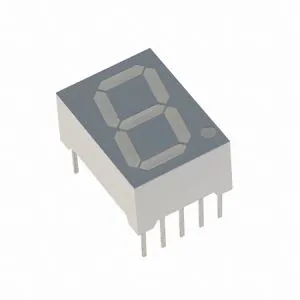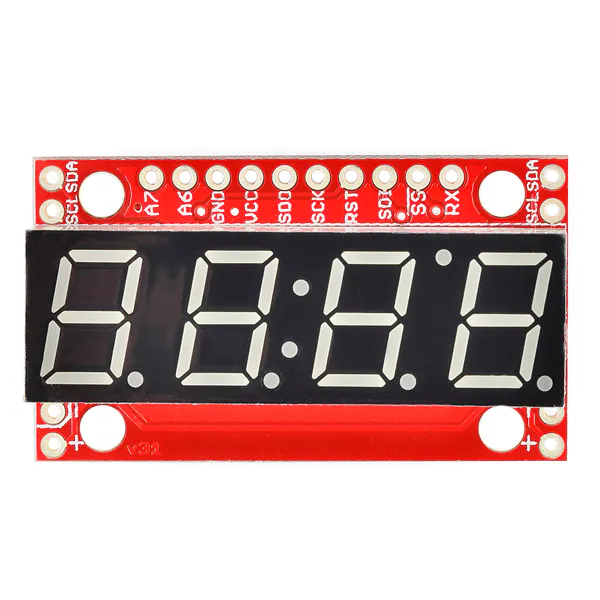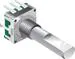# How to Drive a 7 Segment Display with HCF4511

How to drive a seven segment display using only four pins.

BeginnerProtip19 minutes30,379## Things used in this project

### Hardware componentsArduino UNO
×1SparkFun 7-Segment Serial Display - Red
×1
 HCF4511
×1Resistor 10k ohm
×1Rotary Encoder with Push-Button
×1
 470 ohm resistor
×1×1Jumper wires (generic)
×1

## Code

### Code snippet #1

Arduino
```/*
Example of how to drive a seven segment display using HCF4511 or similar

This sketch drive the display in order to show a number from 0 to 9 everytime a button connected to A0 is pressed.

*/

//Declaration of Arduino pins used as HCF4511 inputs
const int A=2;
const int B=3;
const int C=4;
const int D=5;

void setup() {
pinMode(A0, INPUT);
pinMode(A, OUTPUT); //LSB
pinMode(B, OUTPUT);
pinMode(C, OUTPUT);
pinMode(D, OUTPUT); //MSB

}

int count = 0; //the variable used to show the number

void loop() {
if (digitalRead(A0) == LOW) //if button is pressed
{
count++;
delay(200); //the delay prevent from button bouncing
if (count == 10) //we want to count from 0 to 9!
count = 0;
to_BCD(); //convert to binary
}

if (count == 10)
count = 0;

}

void to_BCD()
{
if (count == 0) //write 0000
{
digitalWrite(A, LOW);
digitalWrite(B, LOW);
digitalWrite(C, LOW);
digitalWrite(D, LOW);
}

if (count == 1) //write 0001
{
digitalWrite(A, HIGH);
digitalWrite(B, LOW);
digitalWrite(C, LOW);
digitalWrite(D, LOW);
}

if (count == 2) //write 0010
{
digitalWrite(A, LOW);
digitalWrite(B, HIGH);
digitalWrite(C, LOW);
digitalWrite(D, LOW);
}

if (count == 3) //write 0011
{
digitalWrite(A, HIGH);
digitalWrite(B, HIGH);
digitalWrite(C, LOW);
digitalWrite(D, LOW);
}

if (count == 4) //write 0100
{
digitalWrite(A, LOW);
digitalWrite(B, LOW);
digitalWrite(C, HIGH);
digitalWrite(D, LOW);
}

if (count == 5) //write 0101
{
digitalWrite(A, HIGH);
digitalWrite(B, LOW);
digitalWrite(C, HIGH);
digitalWrite(D, LOW);
}

if (count == 6) //write 0110
{
digitalWrite(A, LOW);
digitalWrite(B, HIGH);
digitalWrite(C, HIGH);
digitalWrite(D, LOW);
}

if (count == 7) //write 0111
{
digitalWrite(A, HIGH);
digitalWrite(B, HIGH);
digitalWrite(C, HIGH);
digitalWrite(D, LOW);
}

if (count == 8) //write 1000
{
digitalWrite(A, LOW);
digitalWrite(B, LOW);
digitalWrite(C, LOW);
digitalWrite(D, HIGH);
}

if (count == 9) //write 1001
{
digitalWrite(A, HIGH);
digitalWrite(B, LOW);
digitalWrite(C, LOW);
digitalWrite(D, HIGH);
}
}
```

## Credits

### Arduino_Scuola

2 projects • 154 followers